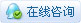1764浏览

# [ 虚谷号入门] 【虚谷号】智能分拣机器人# 1 .原理# 2. 原型设计# 3.实践制作

### 结构搭建### 编写程序

``````import cv2
from xugu import Servo #从 xugu 库中导入 Servo 类
from matplotlib import pyplot as plt
import time
def get_showcampic(fname):
cap = cv2.VideoCapture(0) # 打开摄像头
ret, frame = cap.read()       # 读摄像头
display.clear_output(wait=True) # 设置在一个画面中刷新
cv2.imwrite(fname,frame)
plt.imshow(frame[:,:,::-1])
plt.axis('off') #不显示坐标
plt.show()
cap.release()
cv2.destroyAllWindows()
def get_color(frame):
step=0
red=0
blue=0
green=0
print('开始处理')
high, width, _ = frame.shape
print('剪裁前行数%d，列数%d' % (high, width)) # 裁剪坐标为[x0:x1,y0:y1],截取图像的中心部分
frame=frame[int(high*4/10):int(high*6/10),int(width*4/10):int(width*6/10)]
high, width, _ = frame.shape
print('剪裁后行数%d，列数%d' % (high, width))
#读取图片中所有像素点的RGB值
frame1 = cv2.cvtColor(frame, cv2.COLOR_BGR2RGB)
for i in frame1:
#i中的数据类型是一整行的像素点[[0,0,0][0,0,0]]
step+=1
#读取图片像素点的步长，step==50则为50个像素点读取一次
if (step==20):
for point in i:
#point中的数据类型是单个像素点
red+=point
green+=point
blue+=point
times=0
red=red/len(i)
green=green/len(i)
blue=blue/len(i)
return red,green,blue
while 1:
servo=Servo(4)
servo.write_angle(90)
filename = r"test.jpg"
get_showcampic(filename)
r,g,b=get_color(frame)
if (g>r):
print("更有可能是绿色物品")
servo.write_angle(0)
time.sleep(1)
servo.write_angle(90)
time.sleep(1)
else:
print("更有可能是红色物品")
servo.write_angle(180)
time.sleep(1)
servo.write_angle(90)
time.sleep(1)
print('r:',r)
print('g:',g)
print('b:',b)
time.sleep(5)``````

# 4.功能测试# 6. 参考资料

https://blog.csdn.net/qq_42444944/article/details/89323039

 您需要登录后才可以回帖 登录 | 立即注册 本版积分规则 回帖并转播 回帖后跳转到最后一页

[[wsData.name]]

#### 硬件清单

• [[d.name]]#### 楼主的其它帖子